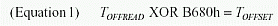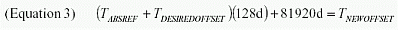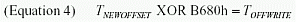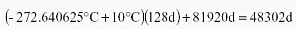### Abstract

The purpose of this application note is to show how to apply a temperature offset to the factory calibrated temperature sensor reading of the DS1859.

### Introduction

The purpose of this application note is to show how to apply a temperature offset to the factory-calibrated temperature sensor reading of the DS1859. The Temperature Offset register should only be modified if the customer's application requires the DS1859 internal temperature reading to have an offset.

### Temperature Offset Register

The Temperature Offset register (Offset Cal Tmp) is located in Table 01h of the Main Device memory at addresses AEh and AFh (see page 21 of the DS1859 data sheet). For each DS1859, the Temperature Offset register is programmed with a value that calibrates the temperature to read 25°C when the IC is exposed to 25°C. It is strongly suggested that value of the factory-calibrated Temperature Offset be recorded and copied into a location in user memory. Once the Temperature Offset register is modified, temperature readings will no longer represent the actual temperature to which the DS1859 is exposed.

### Digitally Applied Temperature Offset

The Temperature Offset is a digitally applied offset to the Temperature Gain (a value that cannot be modified by the user and is a value guaranteed by design). Any changes to the Temperature Offset will be uniformly applied to the Temperature Gain. By adding or subtracting from the Temperature Offset value, a positive or negative offset can be applied to the temperature reading, located in Table 01h at addresses 60h and 61h. Figure 1 shows a block diagram of how the digital Temperature Offset is applied. Modifying the Temperature Offset registers invalidates the factory calibrated temperature reading and digital resistor lookup tables. The Temperature Offset registers should only be modified if there is a need to add an offset to the factory-calibrated temperature reading.### Calculating the Temperature Offset

The first step to calculating a new value for the Temperature Offset is to read the factory-calibrated value from the Temperature Offset register. The value in address AEh is the most significant byte of the Temperature Offset and the value in address AFh is the least significant byte of the Temperature Offset. For the purposes of our calculations, this factory calibrated offset is called TOFFREAD. This value is encoded and must be XOR'd with B680h to attain the actual temperature offset value. The result is called TOFFSET.Once TOFFSET has been calculated, the value must be converted from hex to decimal. This will produce an accurate value for our reference temperature. If a hex value is used for TOFFSET, there will be a loss of temperature accuracy (1°C) when using the remaining equations because the decimal portion of the temperature value will be truncated. Use the following equation to determine the factory-calibrated absolute temperature reference, TABSREF(°C).Once the decimal value of TABSREF has been calculated, the desired temperature offset, TDESIREDOFFSET, can be added or subtracted from TABSREF to find the new temperature offset value, TNEWOFFSET.After the value of TNEWOFFSET has been calculated, it must be converted into hex and then encoded. To encode the new temperature offset, take the hex value of TNEWOFFSET and XOR it with B680h.The value TOFFWRITE should then be written to the Temperature Offset register. In Table 01h, write the most significant byte into address AEh and write the least significant byte into address AFh.

### Temperature Offset Example Calculations

#### Example 1

In the following example, the Temperature Offset register is recalculated so that a DS1859 exposed to 25°C will read 35°C. Therefore, a 10°C offset is desired. Start by reading the factory-calibrated Temperature Offset register. In this example, that register contains 012Eh. Use Equation 1 to XOR this value with B680h to decode the temperature offset value. The result is B7AEh. Converting this hex value into decimal, we get 47022d.Next, use Equation 2 to find the factory-calibrated absolute temperature reference, TABSREF, for this part. In this example, the result is -272.640625°C.Since a 10°C offset is to be added, use Equation 3 to calculate the new temperature offset value, TNEWOFFSET. The result is 48302d.Convert 48302d to hex to get BCAEh. Use Equation 4 to encode this offset so it can be written into the Temperature Offset register. The result is 0A2Eh.In Table 0h, write 0Ah to register AEh and write 2Eh to register AFh to get a +10°C offset.

#### Example 2

In this example, the Temperature Offset register is recalculated so that a DS1859 exposed to 25°C will read 15°C. Therefore, a -10°C offset is desired. To apply a negative offset, follow the same method as stated above to determine the factory calibrated absolute temperature reference (TABSREF = -272.640625°C). For a -10°C offset, use Equation 3 to subtract 10°C from -272.640625°C. The result is 45742d, which is equal to B2AEh.Next, encode the temperature-offset value by using Equation 4.This is the new value to be entered into the Temperature Offset registers (Table 01h, AEh = 04h and AFh = 2Eh) to attain a -10°C offset.

### Conclusion

This application note showed how to apply a positive and negative temperature offset to the factory-calibrated temperature reading of the DS1859. This application note is only intended for customers who require a temperature offset to be applied to the DS1859 temperature sensor reading. Care must be taken to save the factory-calibrated value in the Temperature Offset register of each DS1859 so that those values can be restored should the customer require the original temperature offset. Altering the factory calibrated value for the Temperature Offset will also require the digital resistor lookup tables to be calculated based on the new temperature readings. Questions/comments/suggestions concerning this application note can be sent via Analog document feedback section.